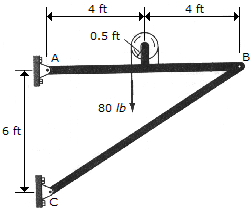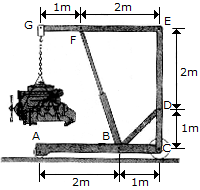# Engineering Mechanics - Structural Analysis

### Exercise :: Structural Analysis - General Questions

• Structural Analysis - General Questions
16.Determine the horizontal and vertical components of force at pin A.

 A. Ax = -46.7 lb, Ay = 45.0 lb B. Ax = -53.3 lb, Ay = 40.0 lb C. Ax = 13.3 lb, Ay = 10.0 lb D. Ax = -106.7 lb, Ay = 80.0 lb

Explanation:

No answer description available for this question. Let us discuss.

17.The hoist supports the 125-kg engine. Determine the force the load creates in member DB and in member FB, which contains the hydraulic cylinder H.

 A. FB = 5.82 kN C, BD = 7.80 kN T B. FB = 1.939 kN C, BD = 2.60 kN T C. FB = 1.839 kN C, BD = 2.47 kN T D. FB = 1.839 kN C, BD = 2.60 kN T